Question

# Arsenic acid is a triprotic acid with the following pKa values: pKa 1 = 2.240; pKa2...

Arsenic acid is a triprotic acid with the following pKa values: pKa 1 = 2.240; pKa2 = 6.960; pKa3 = 11.500. You wish to prepare 1.000 L of a 0.0400 M aresenate buffer at pH 10.430. To do this, you choose to mix the two salt forms involved in the second ionization, Na3AsO4 and Na2HAsO4, in a 1.000 L volumetric flask and add water to the mark. What mass of each salt will you add to the mixture?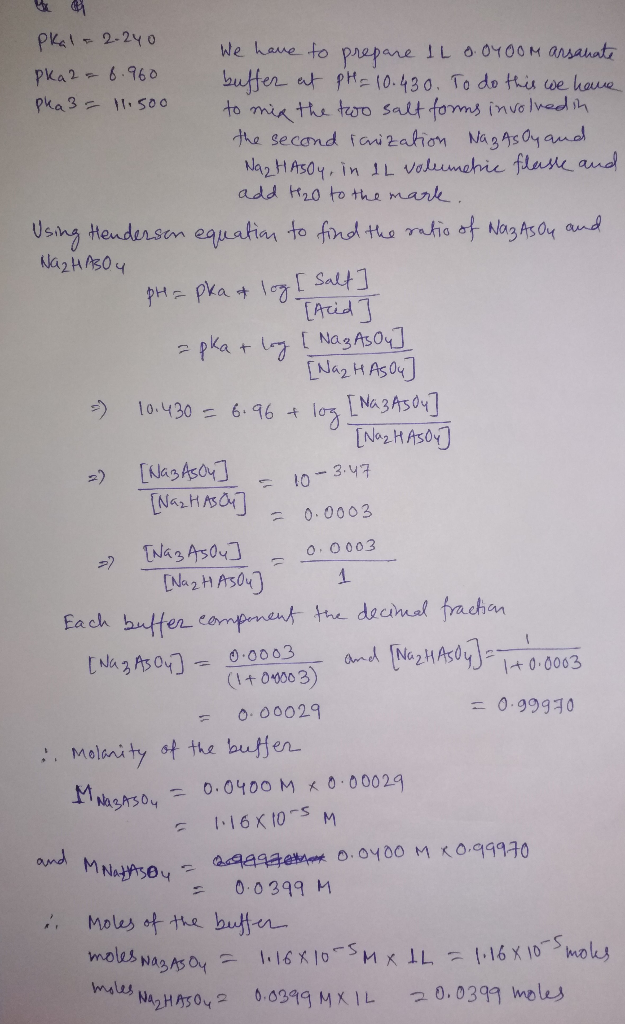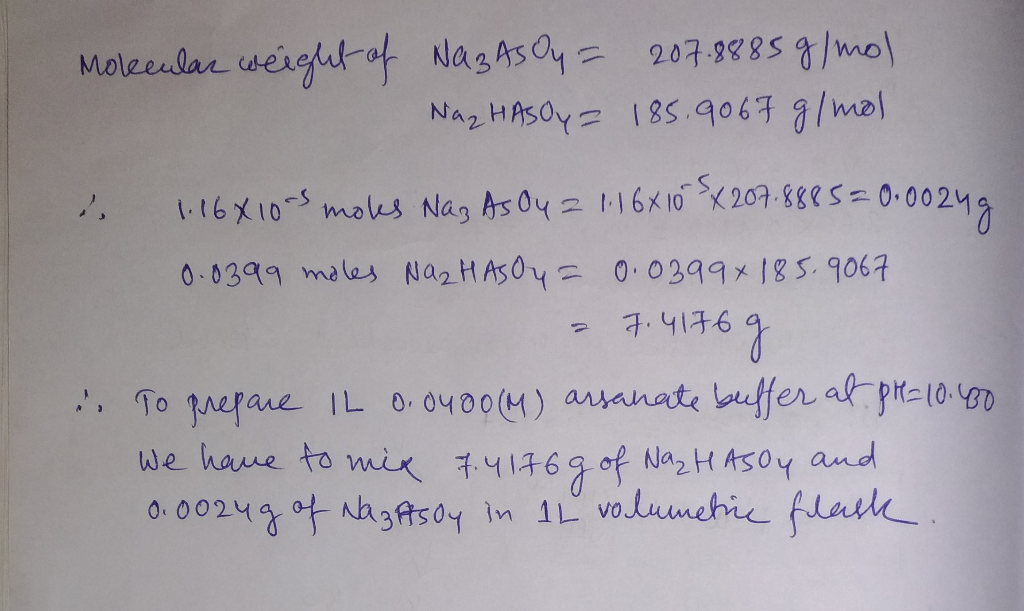#### Earn Coins

Coins can be redeemed for fabulous gifts.

Similar Homework Help Questions
• ### Arsenic acid is a triprotic acid with the pKa values pKal = 2.240, pKa2 = 6.960,...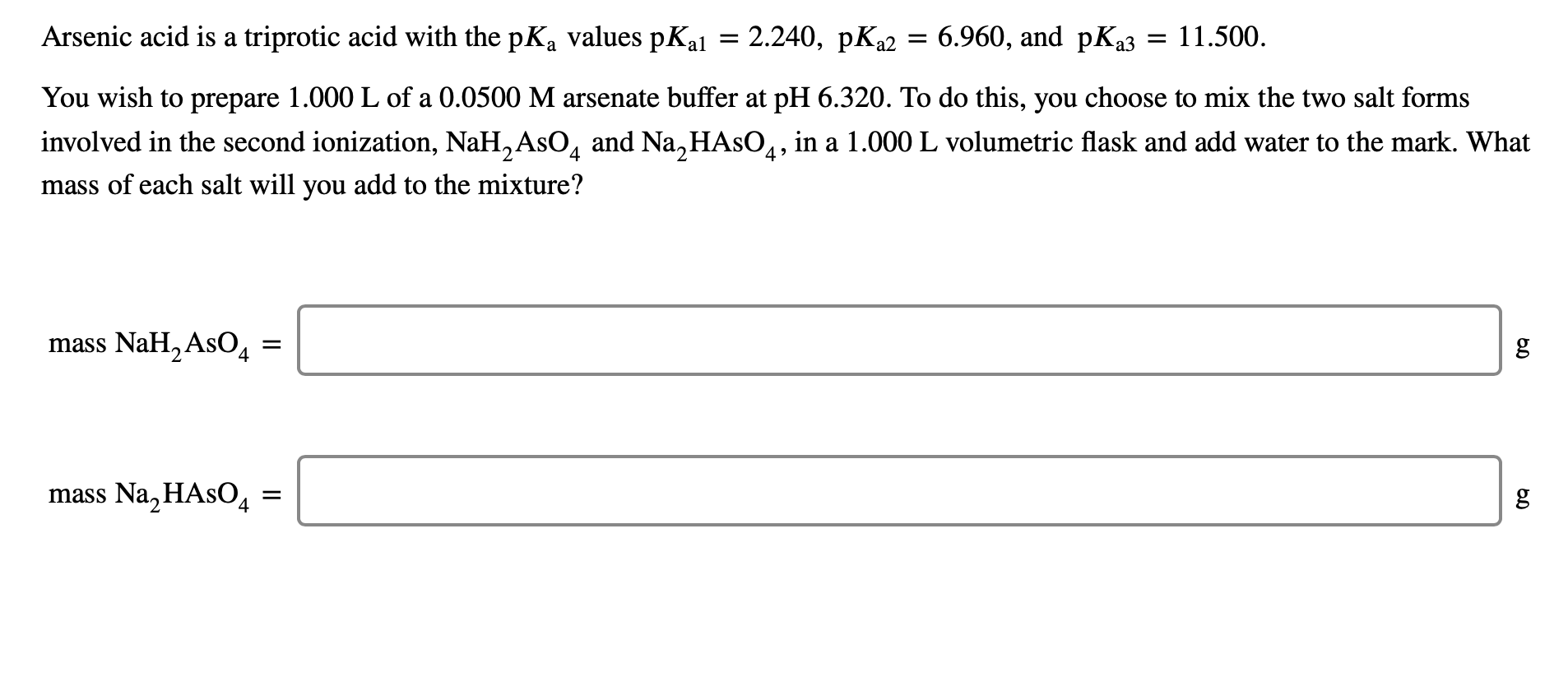Arsenic acid is a triprotic acid with the pKa values pKal = 2.240, pKa2 = 6.960, and pKa3 = 11.500. You wish to prepare 1.000 L of a 0.0500 M arsenate buffer at pH 6.320. To do this, you choose to mix the two salt forms involved in the second ionization, NaH_AsO4 and Na HASO4, in a 1.000 L volumetric flask and add water to the mark. What mass of each salt will you add to the mixture? mass NaH_AsO4...

• ### Phosphoric acid is a triprotic acid with the following pKa values: pKa1=2.148 pKa2=7.198 pKa3=12.375 You wish...

Phosphoric acid is a triprotic acid with the following pKa values: pKa1=2.148 pKa2=7.198 pKa3=12.375 You wish to prepare 1.000 L of a 0.0100 M phosphate buffer at pH 7.580. To do this, you choose to mix the two salt forms involved in the second ionization, NaH2PO4 and Na2HPO4, in a 1.000 L volumetric flask and add water to the mark. What mass of each salt will you add to the mixture? Mass NaH2PO4=? Mass Na2HPO4=?

• ### pling Learning illan learning Phosphoric acid is a triprotic acid with the following pKa values: pKal...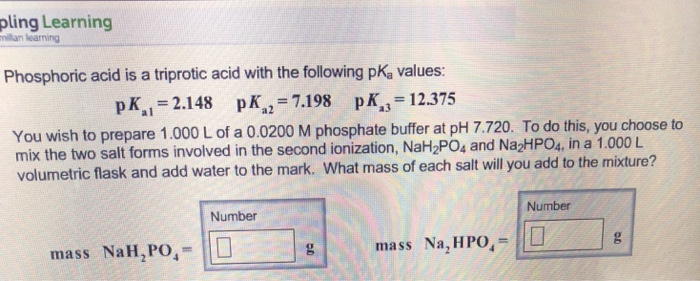pling Learning illan learning Phosphoric acid is a triprotic acid with the following pKa values: pKal = 2.148 pKa,-7.198 pKa3-12.375 You wish to prepare 1.000 L of a 0.0200 M phosphate buffer at pH 7.720. To do this, you choose to mix the two salt forms involved in the second ionization, NaH2PO, and Na2HPO4, in a 1.000 L mass of each salt will you add to the mixture? Number Number mass NaH,PO,-0 mass Na, HPO

• ### Phosphoric acid is a triprotic acid with the pk, values Kal = 2.148, p 2 =...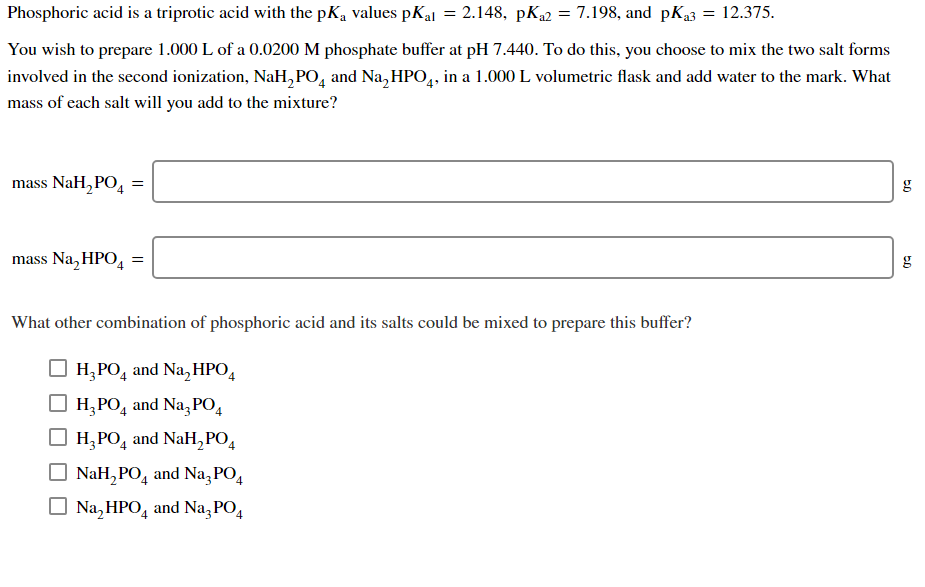Phosphoric acid is a triprotic acid with the pk, values Kal = 2.148, p 2 = 7.198, and pka3 = 12.375. You wish to prepare 1.000 L of a 0.0200 M phosphate buffer at pH 7.440. To do this, you choose to mix the two salt forms involved in the second ionization, NaH, PO, and Na,HPO4, in a 1.000 L volumetric flask and add water to the mark. What mass of each salt will you add to the mixture? mass...

• ### Phosphoric acid is a triprotic acid with the pK, values pK 1 = 2.148, PK 2...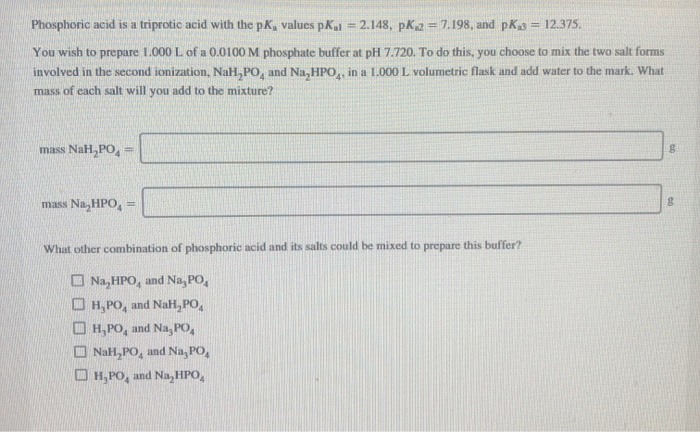Phosphoric acid is a triprotic acid with the pK, values pK 1 = 2.148, PK 2 = 7.198, and pk 3 = 12.375. You wish to prepare 1.000 L of a 0.0100 M phosphate buffer at pH 7.720. To do this, you choose to mix the two salt forms involved in the second ionization, NaH,PO, and Na, HPO,, in a 1.000 L volumetric flask and add water to the mark. What mass of each salt will you add to the...

• ### Phosphoric acid is a triprotic acid with the pK values pK = 2.148, pK2 = 7.198,...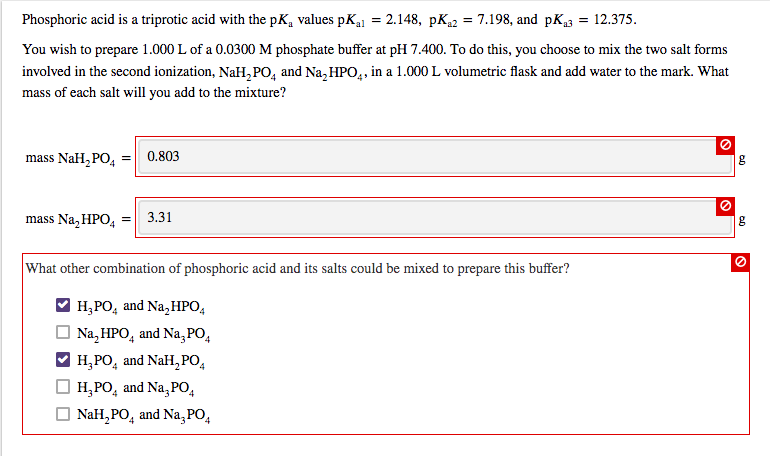Phosphoric acid is a triprotic acid with the pK values pK = 2.148, pK2 = 7.198, and pK3 12.375. You wish to prepare 1.000 L of a 0.0300 M phosphate buffer at pH 7.400. To do this, you choose to mix the two salt forms involved in the second ionization, NaH2 POą and Na, HPO4, in a 1.000 L volumetric flask and add water to the mark. What mass of each salt will you add to the mixture? mass NaH,...

• ### Phosphoric acid is a triprotic acid with the pK, values PK i = 2.148, PK 2...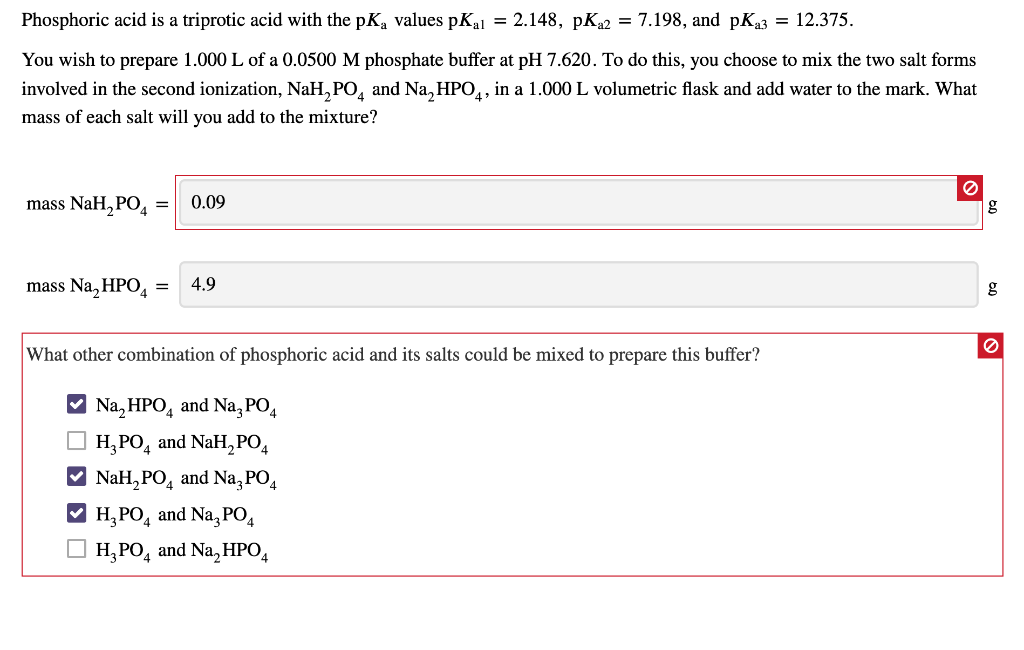Phosphoric acid is a triprotic acid with the pK, values PK i = 2.148, PK 2 = 7.198, and pK23 = 12.375. You wish to prepare 1.000 L of a 0.0500 M phosphate buffer at pH 7.620. To do this, you choose to mix the two salt forms involved in the second ionization, NaH, PO, and Na, HPO4, in a 1.000 L volumetric flask and add water to the mark. What mass of each salt will you add to the...

• ### Phosphoric acid is a triprotic acid with the p?a values p?a1=2.148, p?a2=7.198, and p?a3=12.375. You wish...

Phosphoric acid is a triprotic acid with the p?a values p?a1=2.148, p?a2=7.198, and p?a3=12.375. You wish to prepare 1.000 L of a 0.0300 M phosphate buffer at pH 7.580. To do this, you choose to mix the two salt forms involved in the second ionization, NaH2PO4 and Na2HPO4, in a 1.000 L volumetric flask and add water to the mark. What mass of each salt will you add to the mixture? mass NaH2PO4= g mass Na2HPO4= g What other combination...

• ### Phosphoric acid is a triprotic acid with the pk, values pha=2.148, p 2 7.198, and pks...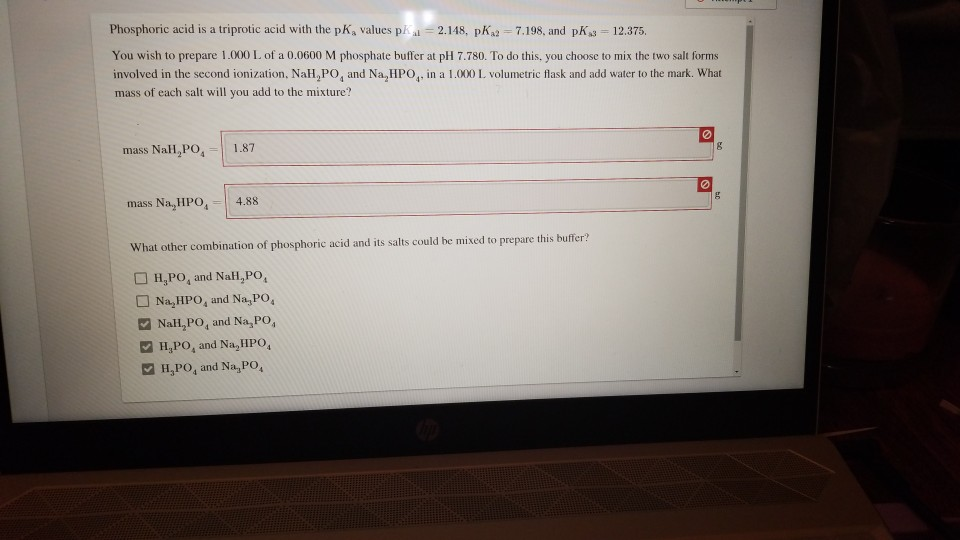Phosphoric acid is a triprotic acid with the pk, values pha=2.148, p 2 7.198, and pks = 12.375. You wish to prepare 1.000 L of a 0.0600 M phosphate buffer at pH 7.780. To do this, you choose to mix the two salt forms involved in the second ionization. Nah,PO, and Na,HPO,, in a 1.000 L volumetric flask and add water to the mark. What mass of each salt will you add to the mixture? mass NaH,PO, 1.87 mass Na,...

• ### For a given triprotic acid H3A, which of its pKa values usually will be the largest?...

For a given triprotic acid H3A, which of its pKa values usually will be the largest? 1. pKa2 2. pKa3 3. pKa1 4. pKa4 5. Not enough information is given. 6. pKa0